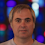## Wednesday, February 29, 2012

### The Erdos- de Bruijn theorem

The mathematician Nicolaas Govert de Bruijn passed away on Feb 17, 2012. The number of things named after him is quite large. I will discuss the Erdos-de Bruijn theorem.

Erdos-de Bruijn theorem: An (infinite) graph G is k-colorable iff every finite subgraph is k-colorable.

I will sketch the proof for the case where G is countable. We can assume the vertices are {1,2,3,...}. Let COLi be a k-coloring of G restricted to {1,...,i}. We will use the COLi's to obtain a k-coloring of the entire graph G. We can assume the COLi's use colors {1,...,k} so we can speak of the least color j such that blah blah.

We color node 1 by the least color that an infinite number of the COLi's color 1. Then REMOVE all of the COLi's that do not use that color on 1. (We kill all those that disagree with us! as I told my students.) Note that there are still an infinite number of COLi's left.

We color node 2 by the least color that an infinite number of the COLi's THAT ARE LEFT color 2. Then REMOVE all of the COLi's that do not use that color on 2. Note that there are still an infinite number of COLi's left.

And so on.

End of Sketch of Proof.
1. This type of argument can be used to proof the following:
1. If we already have the infinite Ramsey Theorem on N, we can obtain the finite Ramsey Theorem and (with a small trick) the Large Ramsey Theorem.
2. If we already have the finite dilworth theorem (any FINITE partial order of width w can be covered with w chains) then we can obtain the infinite version of Dilworth's theorem: if an INFINITE partial order has width w can be covered with w chains.
2. The method is called compactness argument and is very general. It is related to topological compactness, but I won't to into that here. (If a reader has a short explanation or pointer, please post.)
3. The method is noneffective in that if you are given a Turing machine that tells you, for all i,j, COLi(j), then the proof does not appear to be able to give you a Turing machine for a coloring of G. There are two ways this has been formalized, though I list three since the third one strengthens the second one. (For details see my survey of recursive combinatorics here.)
1. (Bean, 1976) There is a computable graph (Vertex set N, Edge set decidable) that is 3-colorable but there is no computable finite coloring whatsoever. (He also made the graph planar, which was not needed but nice.)
2. (Carstens and Pappinghaus, 1983) For every k ≥ 3 There is a highly computable graph (Vertex set N, the function that, given a graph, outputs its finite set of neighbors is computable) that is k-colorable but not computably k-colorable. (NOTE: if a highly comp. graph is k-col then IT IS computably 2k-1 colorable.)
3. (Schmerl, 1980) For every k ≥3 There is a highly computable graph (Vertex set N, the function that, given a graph, outputs its finite set of neighbors is computable) that is k-colorable but not computably (2k-2)-colorable.

1.Alternative proof, works in the uncountable case.

Consider the first order theory that has constants for each node on the graph, k unary predicates, statements that say that precisely one of the k predicates is true at every point, and statements that say for any two adjacent nodes of the graph that the corresponding constants don't have the same predicates true of both. This is consistent because every finite subtheory is consistent, hence by the completeness theorem it has a model. The model is a coloring.

2.I often see such arguments phrased using König's Lemma that every finitely branching tree with an infinite number of nodes has an infinite branch (simple path starting at the root).

In this case, the nodes of the tree are colorings. Level i represents all colorings of {1,...,i}. Node p at level i is a parent of node c at level i+1 if the colorings c and p agree on the colors of all nodes {1,...,i}.

König's Lemma guarantees an infinite branch, which defines a single infinite coloring in the limit.

There is an even shorter way to use compactness arguments that I have seen most often in the literature: "A lot of finite things exist with a property we want. By compactness, an infinite thing with the same property exists. QED"

3.This follows even from compactness of propositional calculus (aka zero-order logic).

You define k variable symbols for each vertex in the graph. You add propositions asserting that exactly one holds for each vertex, as well as propositions for each edge and color.